# math resources

You are currently browsing articles tagged math resources.

## Limits

Some students have asked if there was online material that they could read or study.

• Limits: This follows my explanation of a limit closely. Read examples 1 and 2.
• Left and right handed limits:  Read examples 1 and 3. Practice problems here with solutions.  Remember that the left-handed limit is the limit as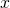approaches the value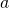from the left-hand side;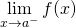. We use thesymbol to denote the left-hand side. The right-handed limit is the limit of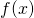asapproaches the valuefrom the right-hand side;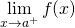. We use thesymbol to denote the right-hand side.
• Limit properties: Read examples 1 and 2.
• Computing Limits: More practice problems with solutions.
• Continuity: Read examples 1 and 2. Practice problems here with solutions (try problems 1 and 2).